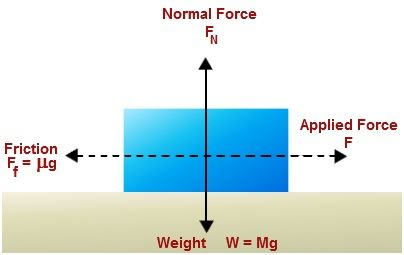# Friction Formula

Friction is the hindering force that comes into play when two items are interacting with each other.Friction formula is typically given as

Ff = μFn

The numeric’s are, the magnitude of friction is Ff , the coefficient of friction is μ  and the magnitude of the normal force is Fn.

The Normal force is equivalent to the weight of the given body. Therefore, the Normal force (Fn) is articulated by:

Fn = mg

Where,
the mass of the object is m and g is the gravity.

The friction formula was made use of to compute the friction between any two given bodies.

Friction Solved Examples

Underneath are problems on friction which helps to know where the formula can be used:

Problem 1: A child is pulling a box of mass 10 Kg. What is the standard force acting and also calculate the frictional force if the coefficient of friction μ is 0.3?

Given: Mass, m = 10 Kg,
Normal force, Fn =?
Normal force is given by Fn = mg
= 10 Kg × 9.8 m/s2
= 98 N.
The Frictional force is articulated by Ff = μ Fn
= 0.3 × 98 N.
= 29.4 N.

Problem  2: Nancy of mass 40 Kg is slipping on the frost. If the coefficient of friction acting is 0.45. Find the frictional force acting between her and the frost layer?